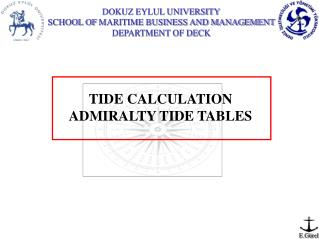DownloadDownload PresentationTélécharger la présentation- - - - - - - - - - - - - - - - - - - - - - - - - - - E N D - - - - - - - - - - - - - - - - - - - - - - - - - - -
Presentation Transcript

1. TIDE CALCULATION ADMIRALTY TIDE TABLES

2. Admiralty Tide Table (ATT) are published in four volumes; • United Kingdom and Ireland (Including English Channel Ports). • Europe (Excluding United Kingdom and Ireland), Mediterranean Sea and Atlantic Ocean. • Indian Ocean and South China Sea (Including Tidal Stream Tables). • Pacific Ocean (Including Tidal Stream Tables).

3. PREDICTED HW RANGE WATER LEVEL HW HEIGHT RISE OF TIDE ( ∆H ) SOUNDING ( DOW) HEIGHT OF TIDE ( H.O.T ) PREDICTED LW LW HEIGHT CHART DATUM CHARTED DEPTH

4. Charted Depth: The depth on a chart is measured from a level known as Chart Datum (CD). On a modern chart, Chart Datum is normally placed at the level of the Lowest Astronomical Tide (LAT) which is the lowest level which can be predicted to occur under average meteorological conditions and under any combination of astronomical conditions. LW and HW Heights: LW and HW heights are always measured from Chart Datum. Rise of Tide (∆H): Rise of Tide is the vertical distance measured from LW to the actual water level. Height of Tide (H.O.T): Height of Tide is the vertical distance measured from Chart Datum to the actual water level.

5. Find the actual sea level, if the C.D is 14,3 m. at 06:20 (GMT) on 1st February 2000. • Duration: HWT – LWT • 10:44 – 03:19 = 7h:25m (Falling) 2. Range: H of HW – H of LW 6,1 – 1,7 = 4,4 m. 3. Δt: HWT – DT or DT – HWT 06:20 – 03:19 = 03h:01m

6. HW 03:19 06:20 Range:4,4 L W ΔH LW 10:44 H of HW: 6,1 H of LW: 1,7 HOT C.D 14,3 SEA BOTTOM DOW = 14,3 + HOT ( ΔH + H of LW )

7. There are two different ways to find the ΔH.

8. First way is to find ΔH = Range x Factor Actual range = 4,4 Δt = 3h:01m After HW Sp 6,0 0,63 Np 3,2 0,59 2,8 0,04 1,2 F F = 0,02 Factor = 0,59 + 0,02 = 0,61 Δh= 4,4 x 0,61 = 2,7 m. DOW = 14,3 + 2,7 + 1,7 = 18,7 m.

9. Second way is to find ΔH with Curves HOT = 4,4 m. Actual range = 4,4 Δt = 3h:01m After HW HW = 6,1 m. LW = 1,7 m. DOW = 14,3 + 4,4 = 18,7 m.

10. 1st February 2000; The sounding taken 17,4 m. by echo-sounder at the noon. If the C.D is 12,7 m. Find the exact time. • Duration: HWT – LWT • 15:43 – 10:44 =4h:59m (Raising) 2. Range: H of HW – H of LW 5,7 – 1,7 = 4,0 m. 3. Δt: HWT – DT or DT – HWT ?????????????????????????????

11. HW 15:43 At noon Range:4 L W ΔH H of HW: 5,7 H of LW: 1,7 LW 10:44 HOT C.D (DOW) Sounding 17,4 12,7 SEA BOTTOM 17,4 = 12,7 + ΔH + 1,7 ; ΔH = 3 m.

12. There are two different ways to find the Δt.

13. First way ΔH = Range x Factor Actual range = 4 3 = 4 x F F = 0,75 Sp 6,0 1h:42m Np 3,2 2h:02m 2,8 20m 0,8 f f = 06m 2h:02m – 06m = 1h:56m 1h:56m Before HW 15h:43m 1h:56m 13h:47m

14. HOT = 4,7 Second way is to find Δt with Curves HOT = 4,7m Actual range = 4 HW = 5,7 m Δt= 1h:56m LW = 1,7 m 1h:56m Before HW 15h:43m 1h:56m 13h:47m

15. THIS PRESANTATION WAS PREPARED BY EVREN GÜREL E MAIL : e.gurel@deu.edu.tr : evrengurel@mynet.com.tr Mobile Phone : 0 532 375 35 31 Phone : 0 232 448 74 34